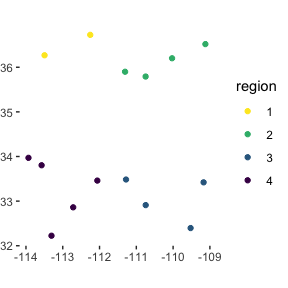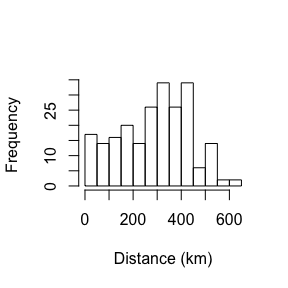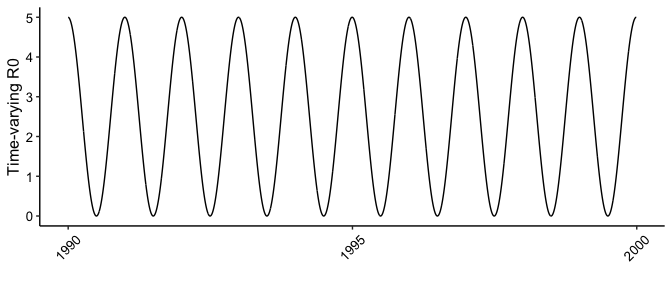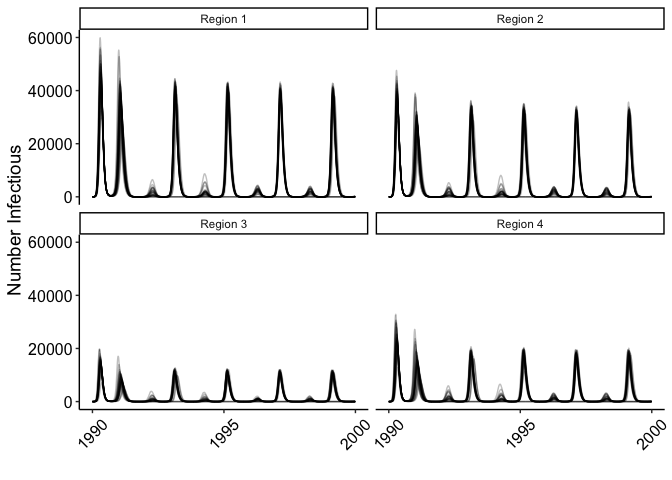# SPARSE-MOD SEIR Model

#### December 2020

library(SPARSEMODr)
library(future.apply)
library(tidyverse)
library(viridis)
library(lubridate)

# To run in parallel:
future::plan("multisession")

## The SEIR Example Model

Here we present a walk-through of using the SPARSE-MOD SEIR Model. SEIR stands for the numbers (not fractions) of Susceptible-Exposed-Infectious-Removed hosts, which define the state variables of the model. The differential equations of this model are: \begin{align} \frac{dS}{dt} &= \overbrace{\mu N}^{\text{Reproduction}} - \overbrace{\hat{\beta} S I}^{\text{Transmission}} - \overbrace{\mu S}^{\text{Natural Mortality}} \\ \frac{dE}{dt} &= \hat{\beta} S I - \overbrace{(\delta + \mu) E}^{\text{Latency and Mortality}} \\ \frac{dI}{dt} &= \delta E - \overbrace{(\gamma + \mu) I}^{\text{Recovery and Mortality}} \\ \frac{dR}{dt} &= \gamma I - \mu R \end{align}

In this classical model, Susceptible individuals become exposed to the pathogen, moving to the Exposed class. There is a period of latency or incubation ($$1/\delta$$) before the hosts become Infectious. Infectious hosts can then recover from the pathogen at an average rate of $$1/\gamma$$, in which case we assume the Recovered individuals are immune to the pathogen for life. The model includes host demography, in which we assume that birth rate is equal to death rate which is equal to $$\mu$$. This ensures that the local population size remains constant through time (not accounting for temporary migration events in the SPARSEMODr framework).1 The model also assumes that all host classes contribute to reproduction and that offspring are fully susceptible. Finally, the model assumes that mortaltity is natural and not pathogen-induced. In other words, the pathogen is not meaningfully virulent in terms of host life-expectancy. This model has been typically used for childhood diseases that confer life-long immunity. Note thta we also use the notation $$\hat{\beta}$$ to emphasize that the transmission rate can be modeled as frequency- or density-dependent.2

In this example, we will demonstrate how we can use the time-windows feature to implement seasonality in transmission rates that can generate sustained oscillations in pathogen prevalence in this SEIR model with host demography.

### Generating a synthetic meta-population

First, we will generate some data that describes the meta-population3 of interest. Note that this is essentially the same set-up as compared to the SPARSEMODr COVID-19 model vignette.

# Set seed for reproducibility
set.seed(2)

# Number of focal populations:
n_pop = 15

# Population sizes + areas
## Draw from neg binom:
pop_N = rnbinom(n_pop, mu = 50000, size = 1)
census_area = rnbinom(n_pop, mu = 50, size = 3)

# Identification variable for later
pop_ID = c(1:n_pop)

# Assign coordinates, plot for reference
lat_temp = runif(n_pop, 32, 37)
long_temp = runif(n_pop, -114, -109)

pop_local_df =
data.frame(pop_ID = pop_ID,
pop_N = pop_N,
census_area,
lat = lat_temp,
long = long_temp) %>%
## Used later for aggregation
mutate(region = case_when(
lat >= 34.5 & long <= -111.5 ~ "1",
lat >= 34.5 & long >  -111.5 ~ "2",
lat <  34.5 & long >  -111.5 ~ "3",
lat <  34.5 & long <= -111.5 ~ "4"
))

# Plot the map:
ggplot(pop_local_df) +
geom_point(aes(x = long, y = lat, color = region),
shape = 19) +
scale_color_viridis_d(direction = -1) +
# Map coord
coord_quickmap() +
theme_classic() +
theme(
axis.line = element_blank(),
axis.title = element_blank(),
plot.margin = unit(c(0, 0.1, 0, 0), "cm")
)

# Calculate pairwise dist
## in meters so divide by 1000 for km
dist_mat = geosphere::distm(cbind(pop_local_df$long, pop_local_df$lat))/1000
hist(dist_mat, xlab = "Distance (km)", main = "")

# We need to determine how many Exposed individuals
# are present at the start in each population
E_pops = vector("numeric", length = n_pop)
# We'll assume a total number of exposed across the
# full meta-community, and then randomly distribute these hosts
n_initial_E = 10
# (more exposed in larger populations)
these_E <- sample.int(n_pop,
size = n_initial_E,
replace = TRUE,
prob = pop_N)
for(i in 1:n_initial_E){
E_pops[these_E[i]] <- E_pops[these_E[i]] + 1
}

pop_local_df$E_pops = E_pops### Setting up the $$\texttt{time_windows}$$ object One of the benefits of the SPARSEMODr design is that the user can specify how certain parameters of the model change over time (see our vignette on key features of SPARSEMODr for more details). We demonstrate this below. In this particular example, we allow the time-varying R0 to change daily in out model, which forces $$\hat{\beta}$$ to fluctuate daily. In this particular example, we apply sinusoidal-forcing, allowing R0 to peak in the fall-winter months and wane in the spring-summer months. We’ll use this particular forcing equation; $R_{0}(t) = \bar{R}_{0} (1 + cos(\frac{2 \pi t}{t_{\text{mode}}}))$ In this case we have an average R0 and $$t_{\text{mode}}$$ controls the number of R0 cycles per year. We’ll use the $$\texttt{time_windows()}$$ function to generate a pattern of time-varying R0 that looks like the following: # Set up the dates of change: # 10 years of day identifiers: n_years = 10 day_ID = rep(c(1:365), times = n_years) date_seq = seq.Date(mdy("1-1-90"), mdy("1-1-90") + length(day_ID) - 1, by = "1 day") # R0 peaks once every how many days? t_mode = 365 # Sinusoidal forcing in R0: r0_base = 2.5 r0_seq = r0_base * (1 + cos((2*pi*day_ID)/t_mode)) # Data frame for plotting: r0_seq_df = data.frame(r0_seq, date_seq) date_breaks = seq(date_seq, date_seq + years(n_years), by = "5 years") ggplot(r0_seq_df) + geom_path(aes(x = date_seq, y = r0_seq)) + scale_x_date(breaks = date_breaks, date_labels = "%Y") + labs(x="", y="Time-varying R0") + # THEME theme_classic()+ theme( axis.text = element_text(size = 10, color = "black"), axis.title = element_text(size = 12, color = "black"), axis.text.x = element_text(angle = 45, vjust = 0.5) )We’ll change the R0, but leave the migration parameters constant. # Set up the time_windows() function n_days = length(date_seq) # Time-varying R0 changing_r0 = r0_seq # Migration rate changing_m = rep(1/10.0, times = n_days) # Migration range changing_dist_param = rep(100, times = n_days) # Immigration (none) changing_imm_frac = rep(0, times = n_days) # Create the time_window() object tw = time_windows( r0 = changing_r0, m = changing_m, dist_param = changing_dist_param, imm_frac = changing_imm_frac, daily = date_seq ) # Create the seir_control() object seir_control = seir_control( input_N_pops = pop_N, input_E_pops = E_pops, birth = 1/(2*365), incubate = 1/5.0, recov = 1/20.0 ) #> Parameter input_I_pops was not specified; assuming to be zeroes. #> Parameter input_R_pops was not specified; assuming to be zeroes. ### Running the spatial SEIR model in parallel Now we have all of the input elements needed to run SPARSEMODr’s SEIR model. Below we demonstrate a workflow to generate stochastic realizations of the model in parallel. Notice that in this host species, breeding (and mortality) occurs on average once every two years. # How many realizations of the model? n_realz = 30 # Need to assign a distinct seed for each realization ## Allows for reproducibility input_realz_seeds = c(1:n_realz) # Run the model in parallel model_output = model_parallel( # Necessary inputs input_dist_mat = dist_mat, input_census_area = pop_local_df$census_area,
input_tw = tw,
input_realz_seeds = input_realz_seeds,
# OTHER MODEL PARAMS
trans_type = 1, # freq-dependent trans
stoch_sd = 0.75,  # stoch transmission sd,
control = seir_control    # data structure with seir-specific params
)

glimpse(model_output)
#> Rows: 1,642,500
#> Columns: 12
#> $pops.seed <int> 1, 1, 1, 1, 1, 1, 1, 1, 1, 1, 1, 1, 1, 1, 1, 1, 1, … #>$ pops.pop          <int> 1, 2, 3, 4, 5, 6, 7, 8, 9, 10, 11, 12, 13, 14, 15, …
#> $pops.time <int> 1, 1, 1, 1, 1, 1, 1, 1, 1, 1, 1, 1, 1, 1, 1, 2, 2, … #>$ pops.S_pop        <int> 3289, 1011, 6182, 5501, 71650, 13455, 80433, 9139, …
#> $pops.E_pop <int> 0, 0, 0, 0, 2, 0, 1, 0, 0, 2, 0, 4, 0, 0, 1, 0, 0, … #>$ pops.I_pop        <int> 0, 0, 0, 0, 0, 0, 0, 0, 0, 0, 0, 0, 0, 0, 0, 0, 0, …
#> $pops.R_pop <int> 0, 0, 0, 0, 0, 0, 0, 0, 0, 0, 0, 0, 0, 0, 0, 0, 0, … #>$ events.birth      <int> 4, 2, 14, 9, 81, 13, 108, 16, 0, 17, 144, 242, 94, …
#> $events.exposed <int> 0, 0, 0, 0, 0, 0, 0, 0, 0, 0, 0, 0, 0, 0, 0, 0, 0, … #>$ events.infectious <int> 0, 0, 0, 0, 0, 0, 0, 0, 0, 2, 0, 2, 0, 0, 1, 0, 0, …
#> $events.recov <int> 0, 0, 0, 0, 0, 0, 0, 0, 0, 0, 0, 0, 0, 0, 0, 0, 0, … #>$ events.death      <int> 2, 2, 8, 2, 94, 19, 118, 11, 0, 17, 137, 189, 125, …

### Plotting the output

First we need to manipulate and aggregate the output data. Here we show an example to plot the number of infectious individuals in the populations over time.

# Grab the new events variables
pops_out_df =
model_output %>%
select(pops.seed:pops.R_pop)

# Simplify/clarify colnames
colnames(pops_out_df) = c("iter","pop_ID","time",
"S", "E", "I", "R")

# Join the region
region_df = pop_local_df %>% select(pop_ID, region)
pops_out_df =
left_join(pops_out_df, region_df, by = "pop_ID")

# Join with dates (instead of "time" integer)
date_df = data.frame(
date = date_seq,
time = c(1:length(date_seq))
)
pops_out_df =
left_join(pops_out_df, date_df, by = "time")

# Aggregate outcomes by region:
## First, get the sum across regions,dates,iterations
pops_sum_df =
pops_out_df %>%
group_by(region, iter, date) %>%
summarize_all(sum)

glimpse(pops_sum_df)
#> Rows: 438,000
#> Columns: 9
#> Groups: region, iter 
#> $region <chr> "1", "1", "1", "1", "1", "1", "1", "1", "1", "1", "1", "1", "1… #>$ iter   <int> 1, 1, 1, 1, 1, 1, 1, 1, 1, 1, 1, 1, 1, 1, 1, 1, 1, 1, 1, 1, 1,…
#> $date <date> 1990-01-01, 1990-01-02, 1990-01-03, 1990-01-04, 1990-01-05, 1… #>$ pop_ID <int> 19, 19, 19, 19, 19, 19, 19, 19, 19, 19, 19, 19, 19, 19, 19, 19…
#> $time <int> 2, 4, 6, 8, 10, 12, 14, 16, 18, 20, 22, 24, 26, 28, 30, 32, 34… #>$ S      <int> 251895, 251964, 251946, 251956, 251920, 251913, 251928, 251936…
#> $E <int> 5, 5, 4, 6, 6, 6, 5, 7, 10, 8, 7, 7, 12, 17, 12, 13, 9, 21, 27… #>$ I      <int> 0, 1, 3, 3, 5, 6, 7, 7, 6, 8, 9, 11, 11, 12, 17, 17, 22, 21, 2…
#> $R <int> 0, 1, 1, 1, 1, 1, 1, 1, 2, 2, 3, 3, 4, 4, 6, 7, 8, 10, 11, 14,… Now we’ll create a simple figure to visualize the stochastic realizations over time.  ####################### # PLOT ####################### # region labels for facets: region_labs = paste0("Region ", sort(unique(region_df$region)))
names(region_labs) = sort(unique(region_df\$region))

# Create an element list for plotting theme:
plot_base =
list(
labs(x = "", y = "Number Infectious"),
theme_classic(),
theme(
axis.text = element_text(size = 12, color = "black"),
axis.title = element_text(size = 14, color = "black"),
axis.text.x = element_text(angle = 45, vjust = 0.5)
)
)

plot_allyears =
ggplot(pops_sum_df) +
geom_path(aes(x = date, y = I, group = iter),
color = "black", alpha = 0.25) +
facet_wrap(~region, scales = "fixed", ncol = 2,
labeller = labeller(region = region_labs)) +
plot_base

plot_allyears1. See our vignette on key features of SPARSEMODr, including migration dynamics for more general details of the SPARSEMODr package.

2. See our vignette on key features of SPARSEMODr, including migration dynamics for more general details of the SPARSEMODr package.

3. A set of distinct, focal populations that are connected by migration